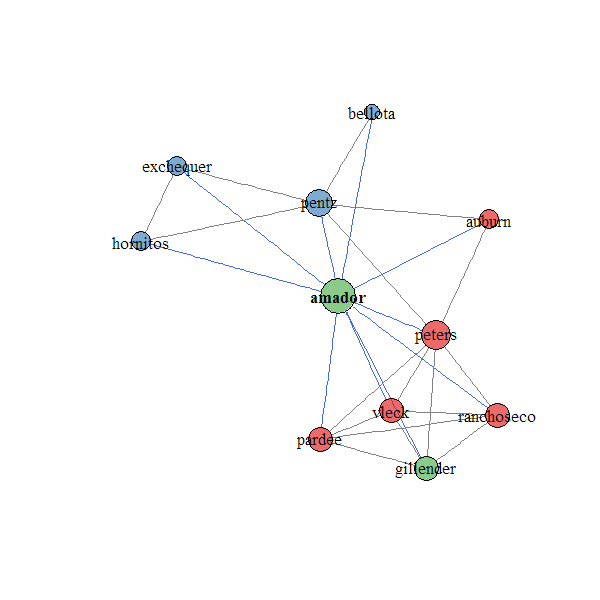component.adj.matrix {sharpshootR} R Documentation

## Create an adjacency matrix from a data.frame of component data

### Description

Create an adjacency matrix from SSURGO component data

### Usage

```component.adj.matrix(d, mu='mukey', co='compname', wt='comppct_r',
method='community.matrix', standardization='max', metric='jaccard',
rm.orphans=TRUE, similarity=TRUE)
```

### Arguments

 `d` a `data.frame`, typically of SSURGO data `mu` name of the column containing the map unit ID (typically 'mukey') `co` name of the column containing the component ID (typically 'compname') `wt` name of the column containing the component weight percent (typically 'comppct_r') `method` one of either: 'community.matrix', or 'occurrence'; see details `standardization` community matrix standardization method, passed to `decostand` `metric` community matrix dissimilarity metric, passed to `vegdist` `rm.orphans` logical, should map units with a single component be ommitted? (typically yes) `similarity` logical, return a similarity matrix? (if FALSE, a distance matrix is returned)

Pending...

### Value

a similatiy matrix / ajacency matrix suitable for use with `igraph` functions or anything else that can accomodate a _similarity_ matrix.

D.E. Beaudette

### Examples

``````# load sample data set